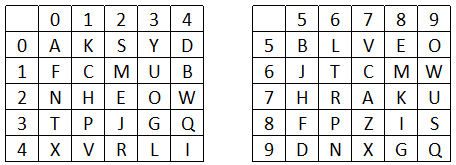Question 3

# A word is represented by only one set of numbers as given in any one of the alternatives. The sets of numbers given in the alternatives are represented by two classes of alphabets as shown in the given two matrices. The columns and rows of Matrix-I are numbered from 0 to 4 and that of Matrix-II are numbered from 5 to 9. A letter from these matrices can be represented first by its row and next by its column, for example, 'C' can be represented by 11, 67, etc., and 'E' can be represented by 22, 58, etc. Similarly, you have to identify the set for the word "FOLK".Solution

(A) : 10, 59, 56, 01 = FOLK

(B) : 85, 59, 43, 79 = FOLU

(C) : 10, 23, 43, 79 = FOLU

(D) : 85, 24, 43, 78 = FWLK

=> Ans - (A)